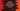# Python program to find the multiplication of all elements in a list## Python program to find the multiplication of all elements in a list :

Python list is one of the most used datatypes. A list can contain an infinite number of items. It the list is empty, it is called an empty list. List items have different datatypes i.e. it can hold elements of string, integer, float or any other types. The items are placed inside a square bracket ([]). All items are separated by a comma.

We can delete or change any element from a list using its index. The index starts from zero for the items, i.e. the index of the first item is zero, the second item is one etc. We can access an element like mylist[i], where _mylist_ is the list and i is the index of the element we are accessing. It will return the item stored in index i.

In this tutorial, we will learn how to multiply all elements of a list in python. First of all, we will add some numbers to a list and then using a for loop we will calculate the multiplication of all elements. Let’s take a look into the program first :

### Python 3 program to multiply all items in a list :

``````#1
my_list = []
#2
for i in range(1,5):
my_list.append(i)
#3
print(my_list)
#4
result = 1
#5
for item in my_list:
result = result * item
#6
print("multiplication of all elements : ",result)``````

You can find the source code here.

### Explanation :

The commented numbers in the above program denote the step number below :

1. Create one empty list mylist_. Here we are using one empty bracket to create this empty list. This is an empty list i.e. list without any items.
2. Add elements to this list using a for loop. The loop will add elements starting from 1 to 4. For appending an element to the list, we are using append() method. This method takes one parameter, i.e. the value that we want to add to the list. In this example, we are adding the current value of i to the list using the append method. The values of i are 1, 2, 3, and 4 on each iteration of the loop. So, the list will be_ [1,2,3,4]_ when the loop ends.
3. Print the list to the user.
4. Declare one variable result as 1. This variable will hold the final multiplication result. We will multiply each element of the list and keep the result in this variable.
5. Run one for loop. This loop will multiply all items of the list and save it in the result variable. This loop iterates each element of the list one by one. On each step, we are multiplying the current element with the result and this value is assigned to result. So, after the loop is completed, the result variable will hold the product of all elements.
6. Finally, print out the result.

### Sample Output :

```````[1, 2, 3, 4]
multiplication of all elements : 24
```````

In this program, we are inserting only four elements to the list to calculate the multiplication. You can add as many items as you want.

### Conclusion :

In this tutorial, we have learned how to find out the product of all items in a python list.

This same process can be used to find out the sum of all items in a list. Or even you can change this program to find out the product of all even or odd indexed items of a list. Try to run the above example and drop one comment below if you have any queries.